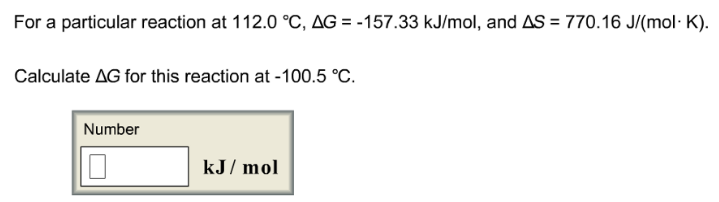# Problem: For a particular reaction at 112.0°C, ΔG = -157.33 kJ/mol, and ΔS = 770.16 J/(mol • K). Calculate ΔG for this reaction at -100.5°C.

###### FREE Expert Solution
97% (293 ratings)###### Problem Details

For a particular reaction at 112.0°C, ΔG = -157.33 kJ/mol, and ΔS = 770.16 J/(mol • K).

Calculate ΔG for this reaction at -100.5°C.